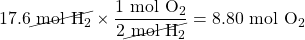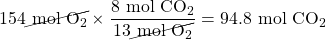Chapter 5. Stoichiometry and the Mole

# The Mole in Chemical Reactions

Learning Objectives

1. Balance a chemical equation in terms of moles.
2. Use the balanced equation to construct conversion factors in terms of moles.
3. Calculate moles of one substance from moles of another substance using a balanced chemical equation.

Consider this balanced chemical equation:

2H2 + O2 → 2H2O

We interpret this as “two molecules of hydrogen react with one molecule of oxygen to make two molecules of water.” The chemical equation is balanced as long as the coefficients are in the ratio 2:1:2. For instance, this chemical equation is also balanced:

100H2 + 50O2 → 100H2O

This equation is not conventional—because convention says that we use the lowest ratio of coefficients — but it is balanced. So is this chemical equation:

5,000H2 + 2,500O2 → 5,000H2O

Again, this is not conventional, but it is still balanced. Suppose we use a much larger number:

12.044 × 1023 H2 + 6.022 × 1023 O2 → 12.044 × 1023 H2O

These coefficients are also in the ratio of 2:1:2. But these numbers are related to the number of things in a mole: the first and last numbers are two times Avogadro’s number, while the second number is Avogadro’s number. That means that the first and last numbers represent 2 mol, while the middle number is just 1 mol. Well, why not just use the number of moles in balancing the chemical equation?

2H2 + O2 → 2H2O

This is the same balanced chemical equation we started with! What this means is that chemical equations are not just balanced in terms of molecules; they are also balanced in terms of moles. We can just as easily read this chemical equation as “two moles of hydrogen react with one mole of oxygen to make two moles of water.” All balanced chemical reactions are balanced in terms of moles.

Example 5.12

# Problem

Interpret this balanced chemical equation in terms of moles.

P4 + 5O2 → P4O10

## Solution

The coefficients represent the number of moles that react, not just molecules. We would speak of this equation as “one mole of molecular phosphorus reacts with five moles of elemental oxygen to make one mole of tetraphosphorus decoxide.”

# Test Yourself

Interpret this balanced chemical equation in terms of moles.

N2 + 3H2 → 2NH3

One mole of elemental nitrogen reacts with three moles of elemental hydrogen to produce two moles of ammonia.

In Chapter 4 “Chemical Reactions and Equations”, in the section called “The Chemical Equation”, we stated that a chemical equation is simply a recipe for a chemical reaction. As such, chemical equations also give us equivalences—equivalences between the reactants and the products. However, now we understand that these equivalences are expressed in terms of moles. Consider the following chemical equation:

2H2 + O2 → 2H2O

This chemical reaction gives us the following equivalences:

2 mol H2 ⇔ 1 mol O2 ⇔ 2 mol H2O

Any two of these quantities can be used to construct a conversion factor that lets us relate the number of moles of one substance to an equivalent number of moles of another substance. If, for example, we want to know how many moles of oxygen will react with 17.6 mol of hydrogen, we construct a conversion factor between 2 mol of H2 and 1 mol of O2 and use it to convert from moles of one substance to moles of another:Note how the mol H2 unit cancels, and mol O2 is the new unit introduced. This is an example of a , when you start with moles of one substance and convert to moles of another substance by using the balanced chemical equation. The example may seem simple because the numbers are small, but numbers won’t always be so simple!

Example 5.13

# Problem

Consider the following balanced chemical equation:

2C4H10(g) + 13O2 → 8CO2(g) + 10H2O(ℓ)

If 154 mol of O2 are reacted, how many moles of CO2 are produced?

## Solution

We are relating an amount of oxygen to an amount of carbon dioxide, so we need the equivalence between these two substances. According to the balanced chemical equation, the equivalence is:

13 mol O2 ⇔ 8 mol CO2

We can use this equivalence to construct the proper conversion factor. We start with what we are given and apply the conversion factor:The mol O2 unit is in the denominator of the conversion factor so it cancels. Both the 8 and the 13 are exact numbers, so they don’t contribute to the number of significant figures in the final answer.

# Test Yourself

Using the above equation, how many moles of H2O are produced when 154 mol of O2 react?

118 mol

It is important to reiterate that balanced chemical equations are balanced in terms of moles. Not grams, kilograms, or litres — but moles. Any stoichiometry problem will likely need to work through the mole unit at some point, especially if you are working with a balanced chemical reaction.

Key Takeaways

• Balanced chemical reactions are balanced in terms of moles.
• A balanced chemical reaction gives equivalences in moles that allow stoichiometry calculations to be performed.

Exercises

# Questions

1. Express in mole terms what this chemical equation means.

CH4 + 2O2 → CO2 + 2H2O

2. Express in mole terms what this chemical equation means.

Na2CO3 + 2HCl → 2NaCl + H2O + CO2

3. How many molecules of each substance are involved in the equation in Exercise 1 if it is interpreted in terms of moles?
4. How many molecules of each substance are involved in the equation in Exercise 2 if it is interpreted in terms of moles?
5. For the chemical equation 2C2H6 + 7O2 → 4CO2 + 6H2O, what equivalences can you write in terms of moles? Use the ⇔ sign.
6. For the chemical equation 2Al + 3Cl2 → 2AlCl3, what equivalences can you write in terms of moles? Use the ⇔ sign.
7. Write the balanced chemical reaction for the combustion of C5H12 (the products are CO2 and H2O) and determine how many moles of H2O are formed when 5.8 mol of O2 are reacted.
8. Write the balanced chemical reaction for the formation of Fe2(SO4)3 from Fe2O3 and SO3 and determine how many moles of Fe2(SO4)3 are formed when 12.7 mol of SO3 are reacted.
9. For the balanced chemical equation 3Cu(s) + 2NO3(aq) + 8H+(aq) → 3Cu2+(aq) + 4H2O(ℓ) + 2NO(g), how many moles of Cu2+ are formed when 55.7 mol of H+ are reacted?
10. For the balanced chemical equation Al(s) + 3Ag+(aq) → Al3+(aq) + 3Ag(s), how many moles of Ag are produced when 0.661 mol of Al are reacted?
11. For the balanced chemical reaction 4NH3(g) + 5O2(g) → 4NO(g) + 6H2O(ℓ), how many moles of H2O are produced when 0.669 mol of NH3 react?
12. For the balanced chemical reaction 4NaOH(aq) + 2S(s) + 3O2(g) → 2Na2SO4(aq) + 2H2O(ℓ), how many moles of Na2SO4 are formed when 1.22 mol of O2 react?
13. For the balanced chemical reaction 4KO2(s) + 2CO2(g) → 2K2CO3(s) + 3O2(g), determine the number of moles of both products formed when 6.88 mol of KO2 react.
14. For the balanced chemical reaction 2AlCl3 + 3H2O(ℓ) → Al2O3 + 6HCl(g), determine the number of moles of both products formed when 0.0552 mol of AlCl3 react.

1. One mole of CH4 reacts with 2 mol of O2 to make 1 mol of CO2 and 2 mol of H2O.
1. 6.022 × 1023 molecules of CH4, 1.2044 × 1024 molecules of O2, 6.022 × 1023 molecules of CO2, and 1.2044 × 1024 molecules of H2O
1. 2 mol of C2H6 ⇔ 7 mol of O2 ⇔ 4 mol of CO2 ⇔ 6 mol of H2O
1. C5H12 + 8 O2 → 5CO2 + 6H2O; 4.4 mol
1. 20.9 mol
1. 1.00 mol
1. 3.44 mol of K2CO3; 5.16 mol of O2